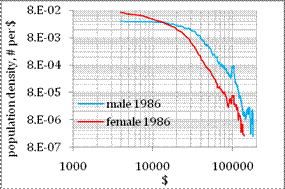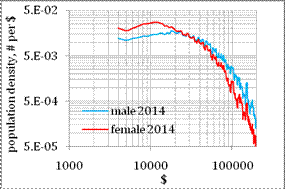## Income And Gender

Personal income distribution for males and females

We first present the change in gender difference related to the distribution of personal income as measured by the Census Bureau. In Figure 9, we compare the male and female population density as obtained in 1986 and 2014. The population density is the ratio of the number of people in a given income bin and the width of this bin. It is measured in the number of people per dollar. When integrated over the entire income range, the curves in Figure 9 give the number of people of a given gender. The IPUMS income microdata data are aggregated in \$1000 bins between \$0 and \$200,000. These income bins are likely too narrow for the 2014 curves and they oscillate over the whole income range, even after smoothing with a MA(7). The same effect is observed at higher incomes, where the number of people is too small and many income bins are just empty. The scarcity of income data at higher incomes has a negative effect of the estimates of the Pareto index.

The level of the female curve is higher at lower incomes. The female population makes approximately 52% of the total working age population, i.e. the number of men and women is approximately equal. Therefore, Figure 9 shows that a larger portion of women has lower incomes. The females’ portion of population with the highest incomes becomes smaller and smaller with growing income. The male-female difference has been likely decreasing with time. In 2014, the males’ curve is closer to the females’ one than in 1986.

The male and female curves intersect at \$14,000 in 1986 and at \$30,000 in 2014 and then the male curve deviates further and further from the female curve. The mean incomes is \$21,822 for men and \$10,741 for women in 1986, in 2014 the mean income is \$53,196 and \$32,588, respectively. The median incomes were \$17,114, \$7,610, \$36,301, and \$22,240, respectively. Therefore, the population density curves intersect near the mean income for females, but the intersection point was above it is 1986 and below in 2014.

Another gender dependent feature, which will be discussed later in this Section, is the income range of the Pareto distribution. In 1986, the straight lines in the log-log scale reveal the Pareto range above \$40,000 for males and above \$30,000 for females. This difference needs special consideration. Essentially, the lower Pareto threshold for women is a strong manifestation of disparity. The meaning of richness is different for females with income. This difference has been improving with time, however, as two curves for 2014 demonstrate.Figure 9. Population density (persons per \$) as a function of income for male and female population in 1986 and 2014. The IPUMS income data are aggregated in \$1000 bins between \$0 and \$200,000. All curves are smoothed by a MA(7) in order to suppress high-amplitude fluctuations. The male and female curves intersect at \$14,000 in 1986 and at \$29,000 in 2014.

Figure 9 also reveals the effect of topcoding. In 1986, the male population in the bin between \$100,000 and \$101,000 is by a factor of 4 larger than that in the adjacent bins. In 2015, the same effect is observed in the bin between \$150,000 and \$151,000, but the factor is 10. For females, there is a deep through preceding the peak at \$100,000 and above. The topcoding may introduce a sizable bias in the estimate of the power law index.

The male and female PIDs in Figure 9 represent aggregated features, and thus, mask the effects of work experience. As we discussed in Section 1, the mean income and especially the evolution of the portion above the Pareto threshold reveal strong dependence on age. The difference in the overall PIDs may also be associated with the change in personal income distribution as a function of age and calendar time, the latter parameter is actually the time series of real GDP per capita. The purpose of the following Subsections is to reveal the difference between genders as expressed in mean income and portion of people above the Pareto threshold and interpret them as linked to the defining parameters of the microeconomic model.

Mean income

The evolution of real mean income (measured in 2014 US\$) for males and females is presented in Figure 10. The male curve is much higher than that for females over the period between 1967 and 2014, where the CPS historical estimates are available. The males’ mean income peaks at \$55,337 in 2000 and then falls to \$51,119 in 2010. The females’ curve peaks at \$33,397 in 2007. This difference indicates that the males’ and females’ PIDs develop independently and react to the overall economic growth in different ways.

Disclosure: None

How did you like this article? Let us know so we can better customize your reading experience. Users' ratings are only visible to themselves.